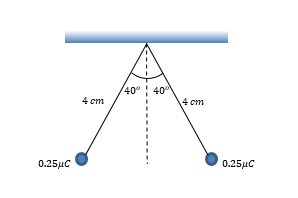# Problem: Two identical,  0.25 μC charges hang from the end of electroscope leaves. If, in equilibrium, the electroscope leaves deflect from one another to an angle of 40° from the horizontal axis, as shown in the figure, what is the mass of each charge?

🤓 Based on our data, we think this question is relevant for Professor Tobolowsky's class at UCLA.

###### Problem Details

Two identical,  0.25 μC charges hang from the end of electroscope leaves. If, in equilibrium, the electroscope leaves deflect from one another to an angle of 40° from the horizontal axis, as shown in the figure, what is the mass of each charge?